# Playing with Numbers

Playing with numbers involves activities like arranging the numbers, understanding BODMAS rule, finding out whether a given number is a factor of another number or a multiple of another number, understanding the properties of factors and multiples. Also, students will learn here, using an index or exponent for a number, simplification of brackets, multiples and factors, divisibility rules from 2 to 11, the concept of co-prime numbers and prime factorization, finding HCF and LCM, etc.,

## Playing with numbers Class 6

Let us learn here how do we play with numbers in Maths based on different concepts. Also, the importance of numbers in our daily life.

### Arranging the objects in rows and columns

 Description Arrangement 1 marble in each row Number of rows = 5 Total number of marbles = 1 × 5 = 5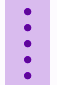2 marbles in each row Number of rows = 4 Total number of marbles = 2 × 4 = 8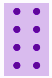6 marble in a row Number of rows = 1 Total number of marbles = 6 (i.e. 6 × 1)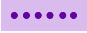### Factors and Multiples of 4

Factors of 4 are the numbers which can divide 4 evenly. It should not be left with any remainder after division. Let us found here the factors.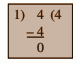Quotient = 4 Remainder = 0 4 = 1 × 4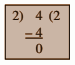Quotient = 2 Remainder = 0 4 = 2 × 2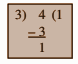Quotient = 1 Remainder = 1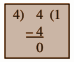Quotient = 1 Remainder = 0 4 = 4 × 1

Hence, the factors of 4 are 1, 2, 4.

Multiples of 4 are equal to 4 times of any natural number. If n is any natural number, then multiples of 4 are given by 4n. Let us find out the multiples here.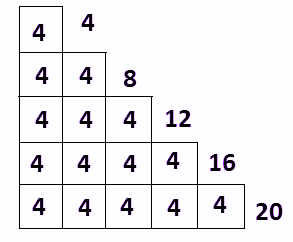Hence, the multiples of 4 are 4, 8, 12, 16, 20,…

The important facts about factors and multiples are:

• Each and every number is a factor of itself
• Each and every factor of a number is an exact divisor of that number
• Each and every factor is less than or equal to the given number
• There are a finite number of factors for a given number
• Each and every multiple of a number is greater than or equal to that number
• There are an infinite number of multiples for a given number
• Every number is a multiple of itself

Perfect number: A number is called a perfect number for which the sum of all its factors is equal to twice the given number.

### Prime and Composite numbers

Prime numbers are the numbers other than 1 whose only factors are 1 and the number itself.

Composite numbers are the numbers having more than two factors.

 Numbers Factors Total factors 1 1 1 2 1, 2 2 3 1, 3 2 4 1, 2, 4 3 5 1, 5 2 6 1, 2, 3, 6 4 7 1, 7 2 8 1, 2, 4, 8 4 9 1, 3, 9 3 10 1, 2, 5, 10 4 11 1, 11 2 12 1, 2, 3, 4, 6, 12 6

In the above table, prime numbers are 2, 3, 5, 7, 11 and the composite numbers are 4, 6, 8, 9, 10 and 12.

Eratosthenes chart of prime numbers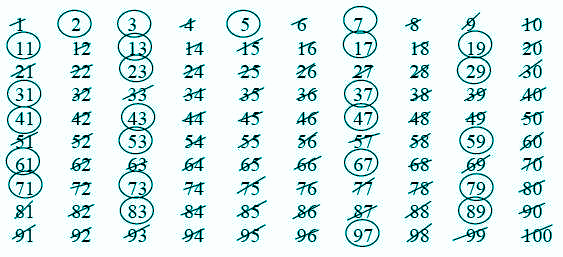The smallest prime number is 2 which is even and except 2 every prime number is odd.

Divisibility Rules

HCF and LCM

Important Questions Class 8 Maths Chapter 16 Playing Numbers

### Factor tree

Factor tree method is used to find the prime factorisation of a given number.

Below is the factor tree of 42: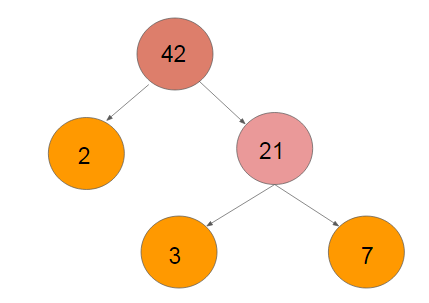Hence, 2, 3, 7 are the prime factors of 42.

## Playing with numbers class 8

General form of numbers:

We can write the two-digit numbers in the general form as shown below.

78 = 10 × 7 + 8

Consider two-digits numbers xy and yx, the general form of these numbers can be written as:

xy = 10 × x + y = 10x+y

yx = 10 × y + x = 10y+b

Similarly, the general form of a three-digit number is:

213 = 100 × 2 + 10 × 1 + 3 = 200 + 10 + 3

xyz = 100 × x + 10 × y + z = 100x+10y+z

Note: Here xy does not represent as multiplication of x and y, but the position of digits in numbers.

## Game with numbers

There are several tricks with numbers to test divisibility by other numbers.

Reversing the digits of a two-digit number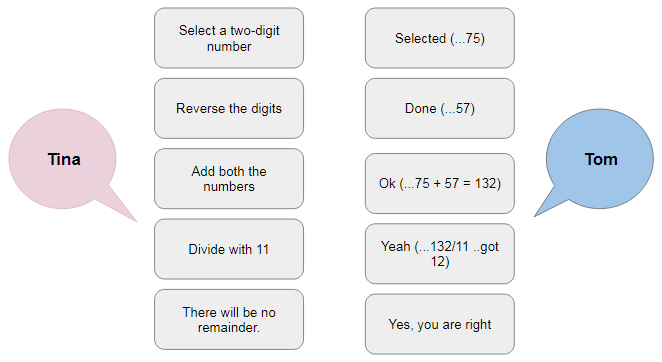Similarly, we can try reversing the digits for dividing by 9.

Selecting a two-digit number: 25

Reversing the digits: 52

Subtract: 52-25 = 27

Divide by 9: 27/9 = 3

Remainder is 0.

Reversing the digits of a three-digit number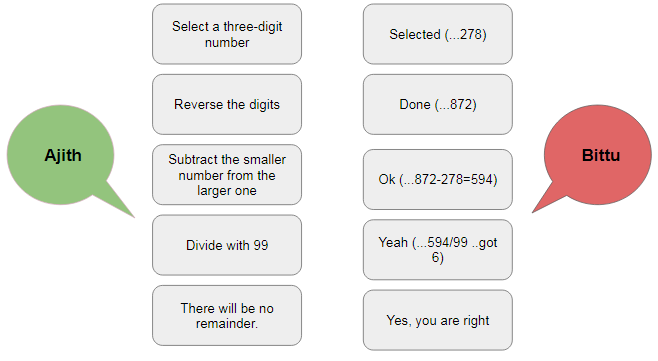Forming three-digit number from the given three digits

For a given three-digit number, rearrange the digits such that all the three numbers are distinct and add them all. Then dividing it by 37, the remainder is always 0.

For example, assuming a three-digit number 124.

On rearranging the digits: 241 and 412.

Add all the three numbers: 124 + 241 + 412 = 777

Divide 777 by 37: 777/37 = 21; remainder is 0.

### Letters of digits

There are some puzzles in which letters take the place of digits in an arithmetic ‘sum’, and the problem is to find out which letter represents which digit; so it is like cracking a code. Here are some problems of addition and multiplication. Two major rules of this kind of puzzles are:

Each digit is to represent a single letter and vice versa.

The number should not contain 0 as its first digit. For example, 45 should not be given as 045 or 0045.

Find the value of R in the below addition.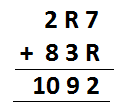In the above addition, 7 + R giving “2” that means the unit digit of the sum is 2. This is possible only if R = 5.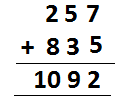Puzzles with Multiplication

Find the value of A and B in the below multiplication.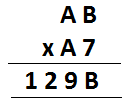B × 7 = B, it is possible if B = 0 or B = 5

If A = 1 and B = 0, AB × A7 = 10 × 17 = 170

If A = 1 and B = 5, AB × A7 = 15 × 17 = 255

If A = 2 and B = 0, AB × A7 = 20 × 27 = 540

If A = 2 and B= 5, AB × A7 = 25 × 27 = 675

If A = 3 and B= 0, AB × A7 = 30 × 37 = 1110

If A = 3 and B = 5, AB × A7 = 35 × 37 = 1295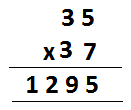Test your Knowledge on Playing with Numbers